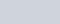The first term of an A.P. is 2 and the last term is 50.
Question:

The first term of an A.P. is 2 and the last term is 50. The sum of all these terms is 442. Find the common difference.

Solution:

In the given problem, we have the first and the last term of an A.P. along with the sum of all the terms of A.P. Here, we need to find the common difference of the A.P.

Here,

The first term of the A.P (a) = 2

The last term of the A.P (l) = 50

Sum of all the termsLet the common difference of the A.P. be d.

So, let us first find the number of the terms (n) using the formula,

$442=\left(\frac{n}{2}\right)(2+50)$

$442=\left(\frac{n}{2}\right)(52)$

$442=(n)(26)$

$n=\frac{442}{26}$

$n=17$

Now, to find the common difference of the A.P. we use the following formula,

$l=a+(n-1) d$

We get,

$50=2+(17-1) d$

$50=2+17 d-d$

$50=2+16 d$

$\frac{50-2}{16}=d$

Further, solving for d,

$d=\frac{48}{16}$

$d=3$

Therefore the common difference of the A.P $d=3$.